# Create And Modify Formulas

In Crystal Report, It is very easy to create and modify formulas. When we create formulas in our report, it makes our all calculation easy and fast. Once we create this in the report, we can use this again and again. We can also delete and modify the formulas, according to our conditions.

Create Formulas

To create a new formula, Right click on the formula tab under Field Explorer → New. When we click New tab, it gives an option 'Formula Name'.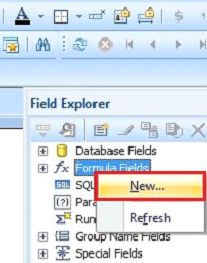Here, give the formula name and click OK.We can easily find our formula name by expanding the 'Formula Fields'.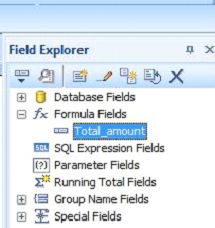After completing all the above process, we find a window on our screen, as shown below. Here, we see our report fields, formula name, formula functions, formula operators etc.

To write the formula, select and double click on the report fields, as shown in the image. Subsequently, we choose an operator, which we apply on the formulas.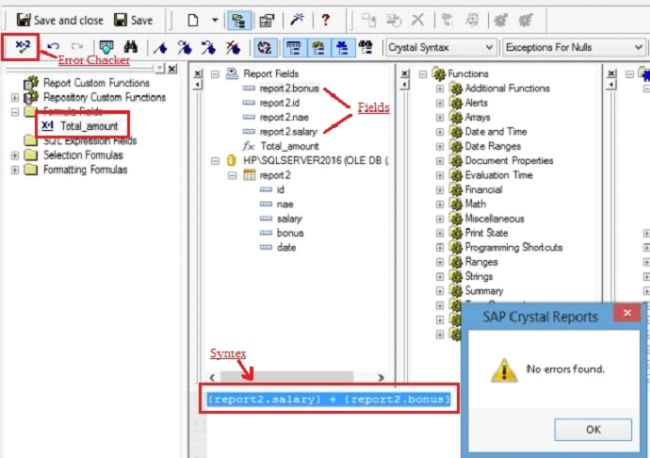We can save the formula, using the save option at the bottom of the page. Also check an error with the 'Error Checker' tab in the formula workshop. We can drag this formula to any section of the report.

Now, see the output. Go to Design in our report and drag the 'Formulas Name' by 'Field Explorer'.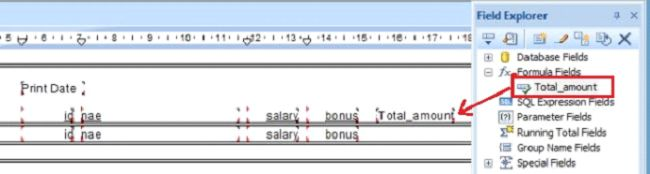Go to Preview and see our output.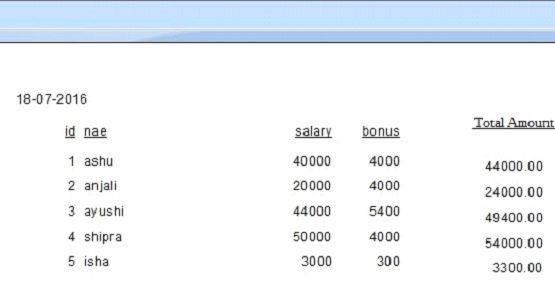Modify Formulas

To modify the formula, click formula name in Field explorer. It will open the formula workshop. Here, we can easily change our formula and save the changes.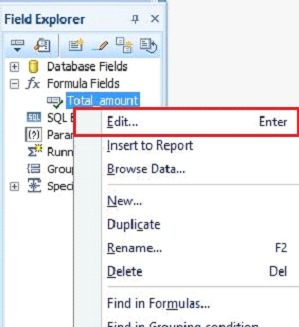Delete Formulas

We can also delete the formula, Right click on the formula name in the 'Field Explorer' or inside the 'Formula Workshop' and click Delete.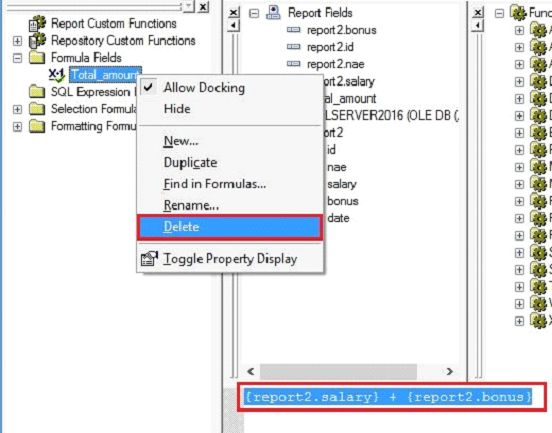Summary

Thus, we learnt to create and modify the formulas in Crystal Report because it makes the calculation easy and fast.
Next » Formulas With ‘Boolean Operators’